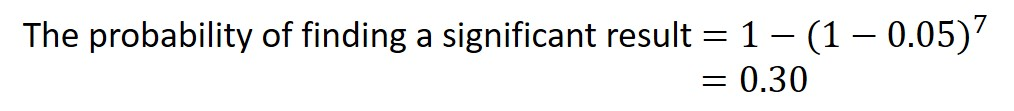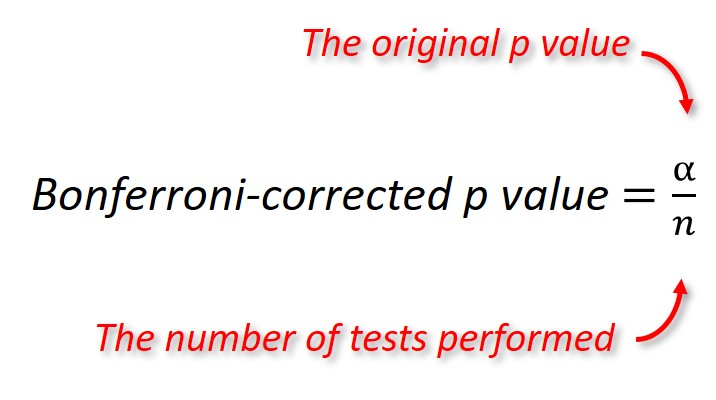# The Bonferroni Correction Method Explained

In this guide, I will explain what the Bonferroni correction method is in hypothesis testing, why to use it and how to perform it.

## What is the Bonferroni correction method?

Simply, the Bonferroni correction, also known as the Bonferroni type adjustment, is one of the simplest methods use during multiple comparison testing. Named after its Italian curator, Carlo Emilio Bonferroni, the Bonferroni correction method is used to compensate for Type I error.

## What is Type I error?

Type I error, in the context of hypothesis testing, is the likelihood of discovering a false-positive result, thus rejecting a true null hypothesis.

Usually, the Type I error rate (the alpha level) in hypothesis testing is set to 5% (ie the p-value is 0.05). This means that 5% of the time, you are willing to accept a false-positive result.

However, when there are multiple comparisons being made, the type I error rate will rise. For example, let’s say there is a hypothesis with 7 comparisons being performed – what is the probability of discovering a false-positive result?Therefore, instead of a 5% rate, the Type I error rate is now 30%. In other words, in this situation, there is a 30% chance of discovering a false-positive result.

Now you can understand the importance of controlling for multiple comparisons.

## The Bonferroni correction method formula

The Bonferroni correction method is regarding as the simplest, yet most conservative, approach for controlling Type I error.

To perform the correction, simply divide the original alpha level (most like set to 0.05) by the number of tests being performed.The output from the equation is a Bonferroni-corrected p value which will be the new threshold that needs to be reached for a single test to be classed as significant.

## A Bonferroni correction example

Let’s say we have performed an experiment whereby a group of young and old adults were tested on 5 memory tests. We are now interested in determining if any of these memory test scores differ between the two age groups. Since all 5 memory tests are essentially measuring the same outcome, we will need to apply multiple comparison corrections to control for Type I error.

The first thing we need to do is to create a new Bonferroni-correct p value to take into account the multiple testing.

To do this, I will divide the original p value (0.05) by the number of tests being performed (5). Doing so will give a new corrected p value of 0.01 (ie 0.05/5).

Below is a table containing some more examples of the number of multiple tests and the new Bonferroni-correct p values associated with them. Note, these assume the original p value was 0.05.

 Number of tests Bonferroni-corrected p value 2 0.025 3 0.017 4 0.0125 5 0.01 10 0.005 15 0.003 20 0.0025

## Alternatives to the Bonferroni correction method

Since the Bonferroni correction method is rather strict, it may be better suited to use less-conservative methods when controlling for Type I error.

Below is a list of some alternatives to the Bonferroni correction method.

• Sidak method
• Tukey method
• Holm-Bonferroni method
• Hochberg method
• Dunnett method

## Conclusion

In sum, the Bonferroni correction method is a simple way of controlling the Type I error rate in hypothesis testing. To calculate the new alpha level, simply divide the original alpha by the number of comparisons being made. However, since this approach is rather strict, it may be more appropriate to use alternative means of controlling for multiple comparisons.